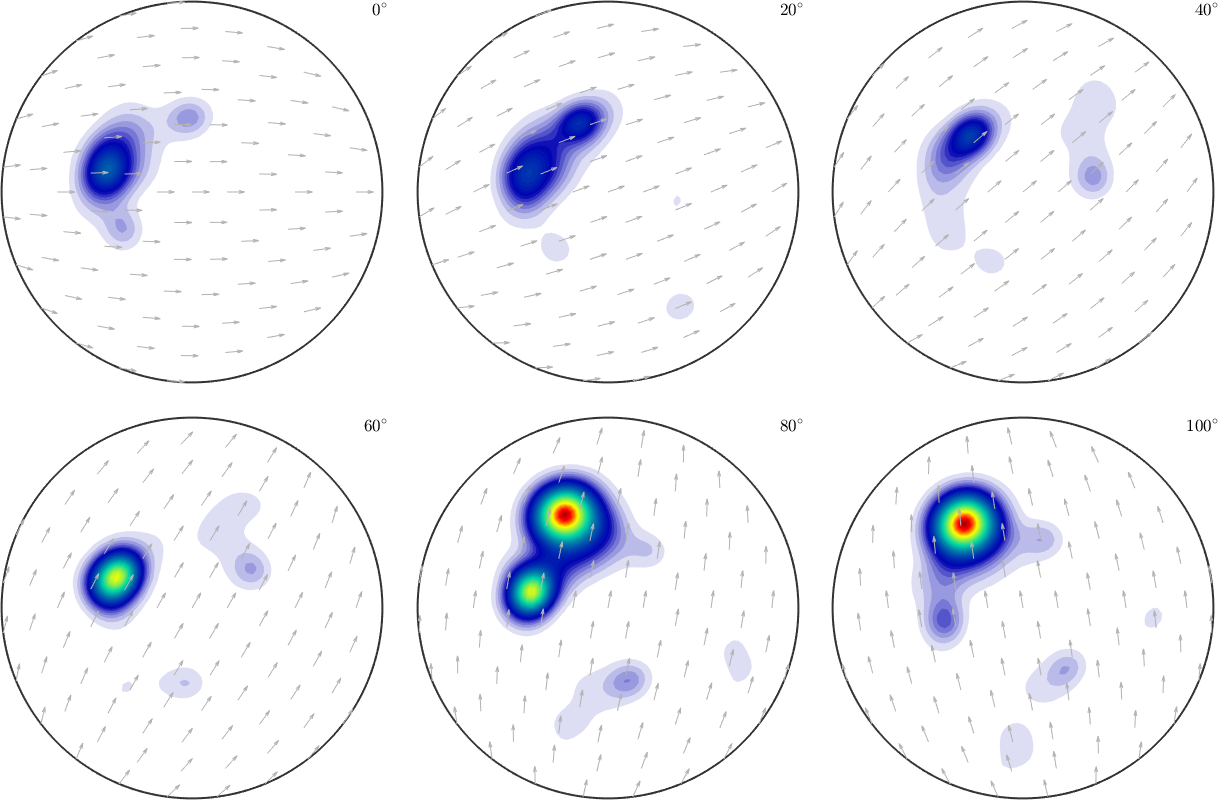Operations on Rotational Functions edit page

The idea of variables of type SO3Fun is to calculate with rotational functions similarly as Matlab does with vectors and matrices. In order to illustrate this we consider the following two rotational functions

An ODF determined from XRD data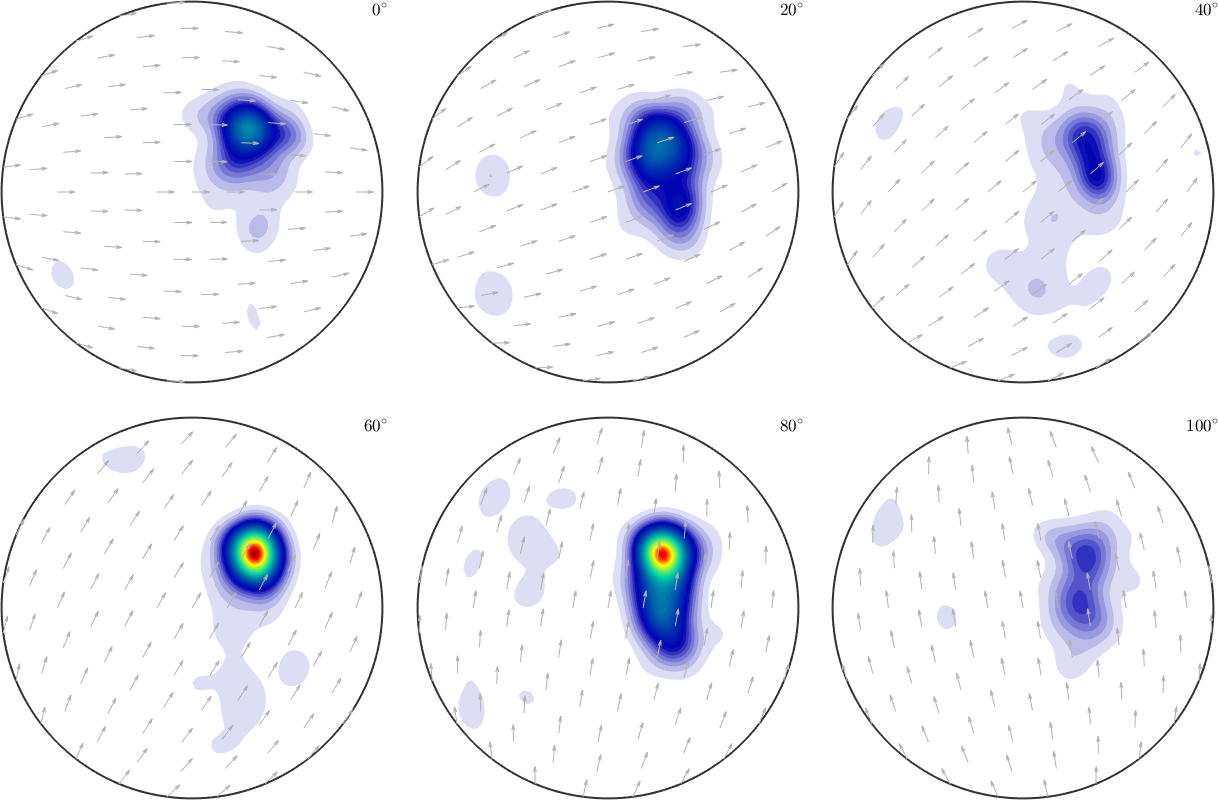and an unimodal distributed ODF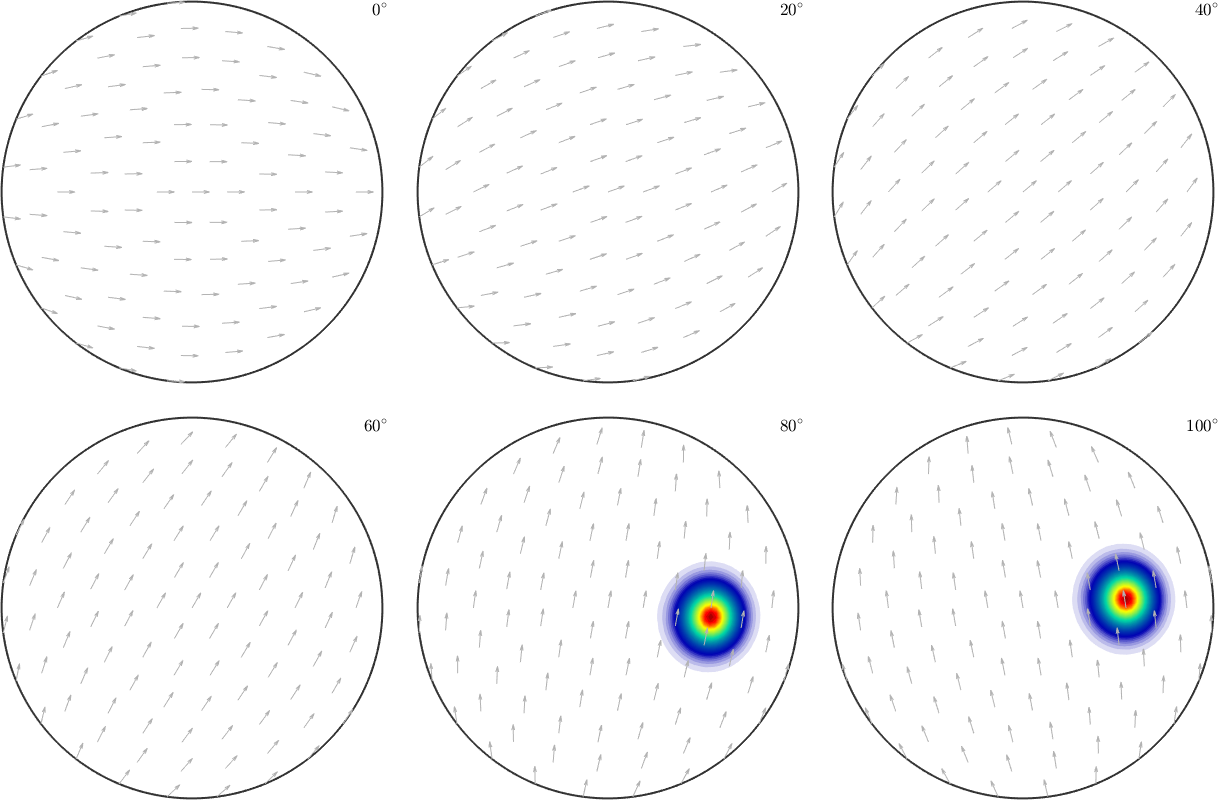## Basic arithmetic operations

Now the sum of these two rotational functions is again a rotational function, i.e., a function of type SO3Fun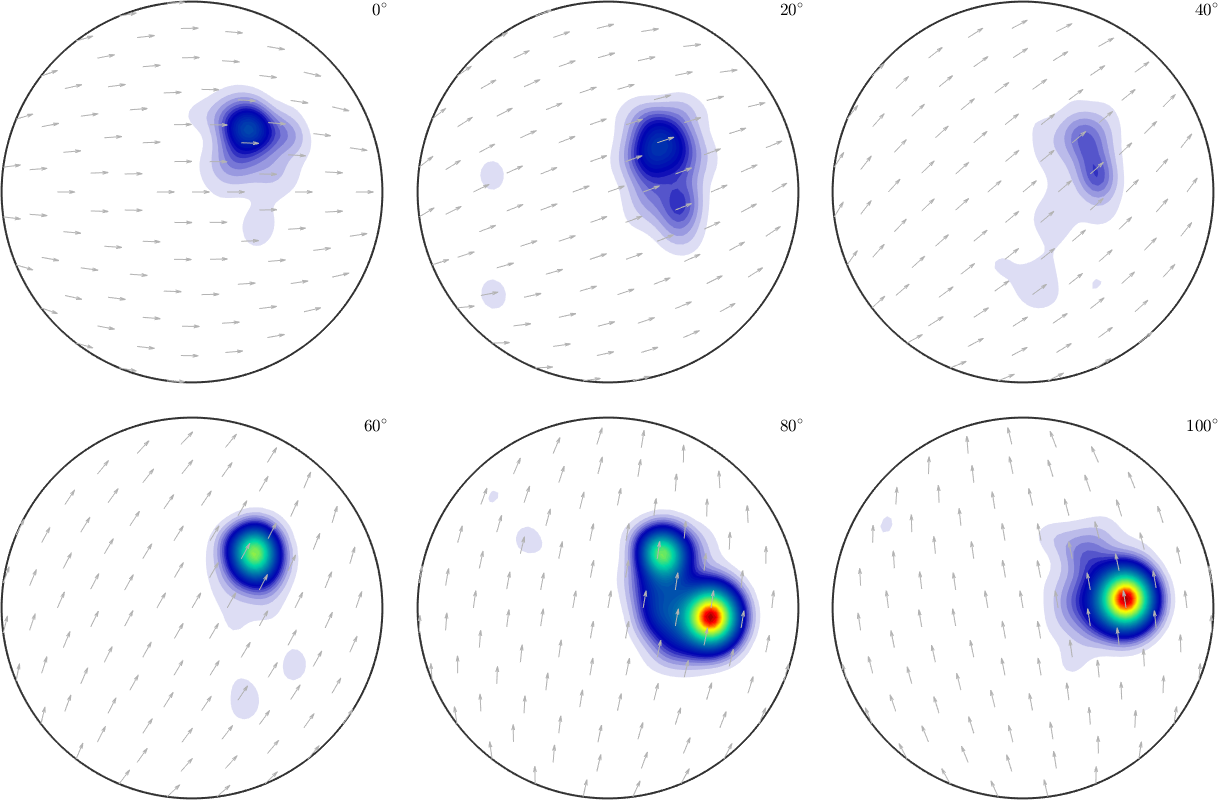Accordingly, one can use all basic operations like -, *, ^, /, min, max, abs, sqrt to calculate with variables of type SO3Fun.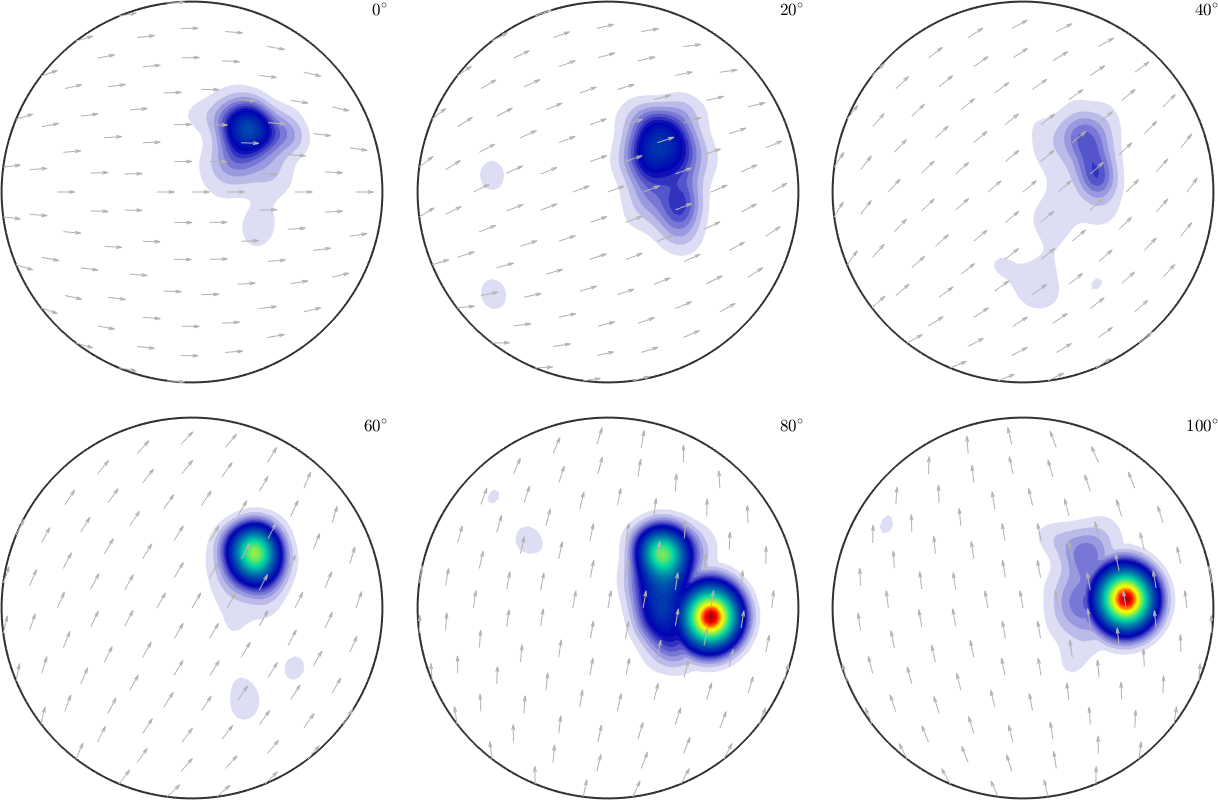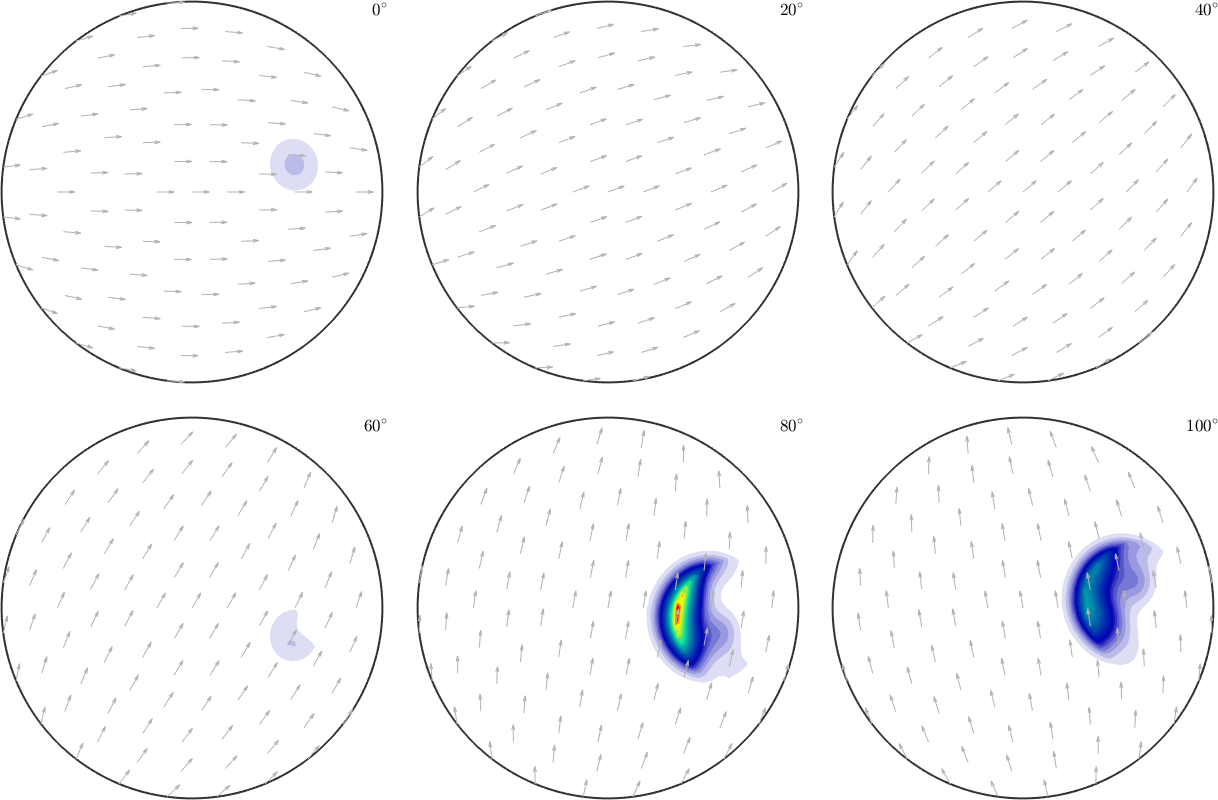We also can work with the pointwise conj, exp or log of an SO3Fun.

For a given function $$f\colon SO(3) \to \mathbb C$$ we get a second function $$g\colon SO(3) \to \mathbb C$$ where $$g( {\bf R}) = f( {\bf R}^{-1})$$ by the method inv, i.e.

## Local Extrema

The above mentioned functions min and max have very different use cases

• if a single rotational function is provided the global maximum / minimum of the function is computed
• if two rotational functions are provied, a rotational function defined as the pointwise min/max between these two functions is computed
• if a rotational function and a single number are passed as arguments a rotational function defined as the pointwise min/max between the function and the value is computed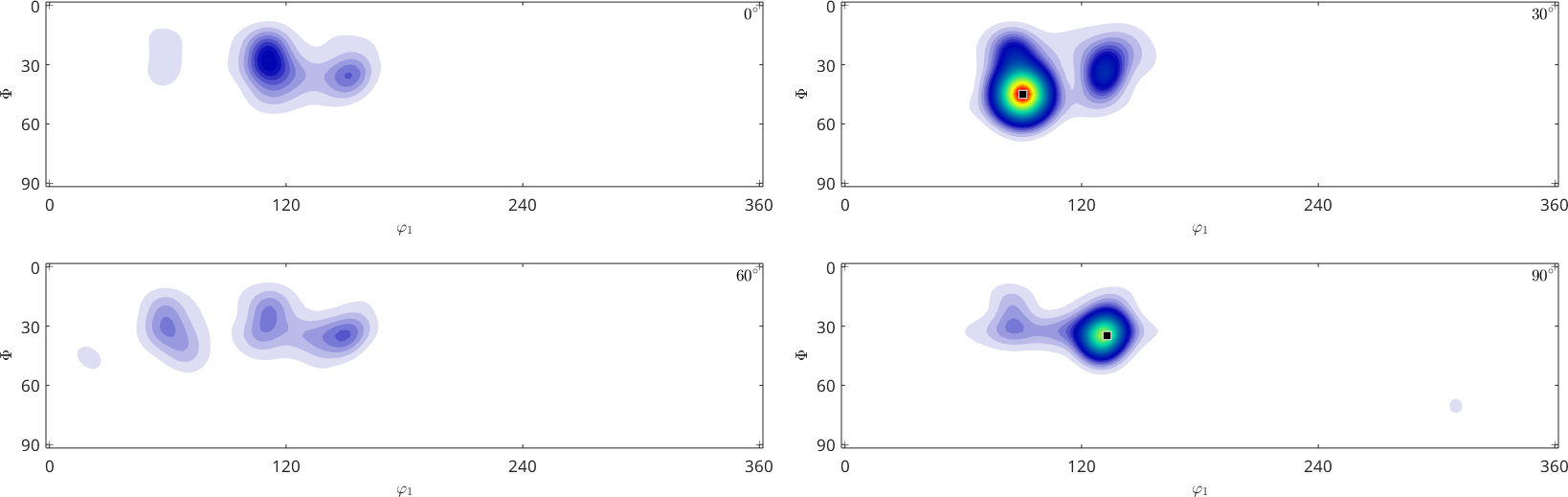## Integration

The surface integral of a spherical function can be computed by either mean or sum. The difference between both commands is that sum normalizes the integral of the identical function on the rotation group to $$8 \pi^2$$, the command mean normalizes it to one. Compare

A practical application of integration is the computation of the $$L^2$$-norm which is defined for a $$SO(3)$$ function $$f$$ by

$\| f\|_2 = \left( \frac{1}{8\pi^2} \int_{SO(3)} \lvert f({\bf R}) \rvert^2 \,\mathrm d {\bf R} \right)^{1/2}$

accordingly we can compute it by

or more efficiently by the command norm

## Differentiation

The gradient of a $$SO(3)$$ function in a specific point can be described by a three-dimensional vector which can be computed by the command grad

The gradients of a $$SO(3)$$ function in all points form a $$SO(3)$$ vector field and are returned by the function grad as a variable of type SO3VectorFieldHarmonic.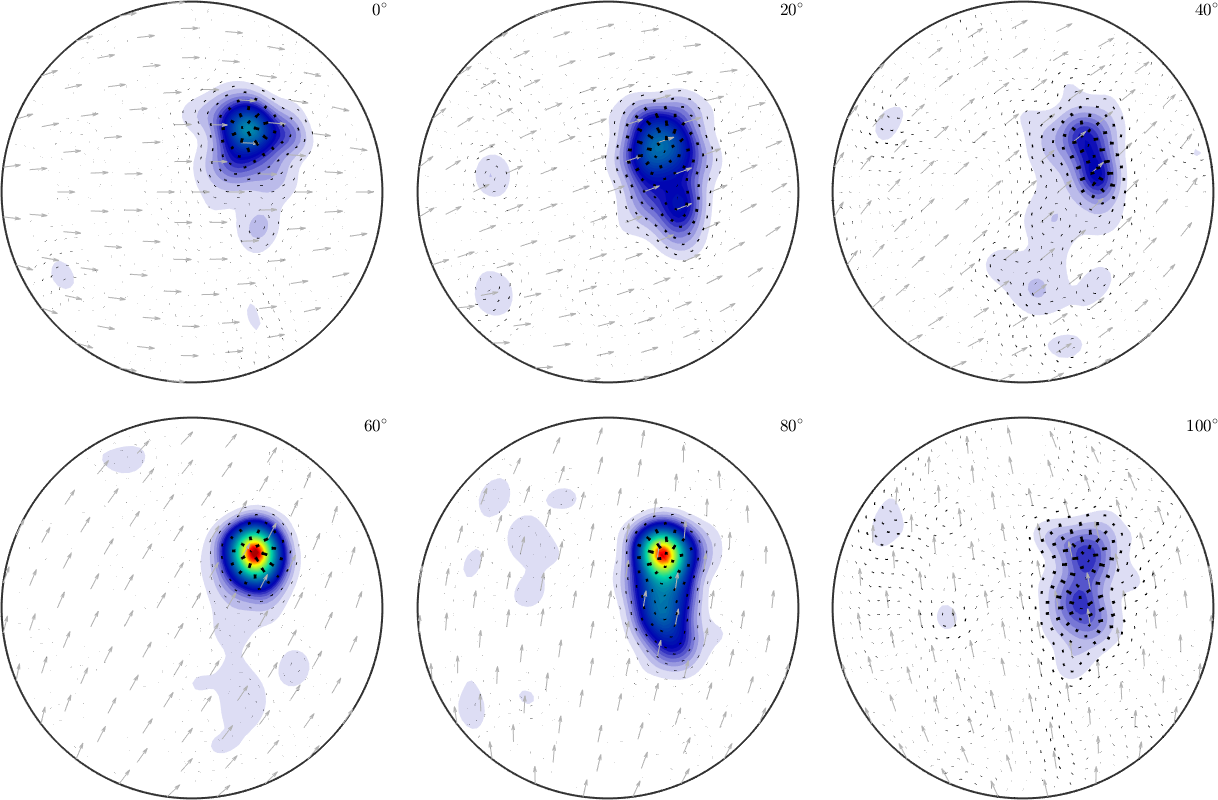We observe long arrows at the positions of big changes in intensity and almost invisible arrows in regions of constant intensity.

## Rotating rotational functions

Rotating a $$SO(3)$$ function works with the command rotate